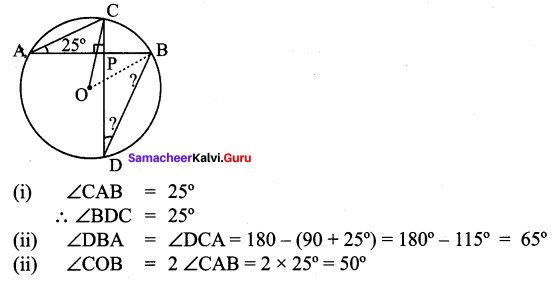## Tamilnadu Samacheer Kalvi 9th Maths Solutions Chapter 4 Geometry Ex 4.3

Question 1.
The diameter of the circle is 52 cm and the length of one of its chord is 20 cm. Find the distance of the chord from the centre.
Solution:
The distance of the chord from the centre O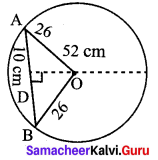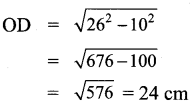Question 2.
The chord of length 30 cm is drawn at the distance of 8cm from the centre of the circle. Find the radius of the circle.
Solution: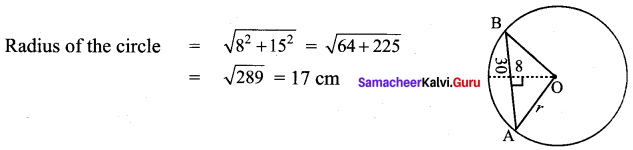Question 3.
Find the length of the chord AC where AB and CD are the two diameters perpendicular to each other of a circle with radius 4 $$\sqrt{2}$$ cm and also find ∠OAC and ∠OCA.
Solution:
∆OAC is an isoceles triangle with one angle 90°
∴ ∠OAC + ∠OCA = 180° – 90°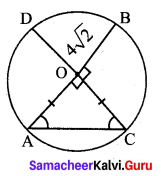2∠OAC = 90°
∠OAC = 45°
∴ ∠OCA = 45°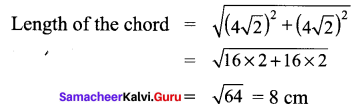Question 4.
A chord is 12cm away from the centre of the circle of radius 15 cm. Find the length of the chord.
Solution: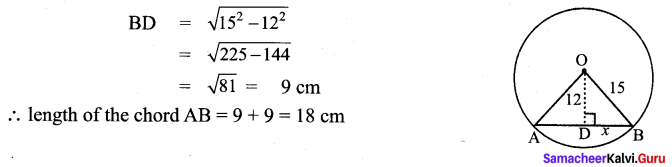Question 5.
In a circle, AB and CD are two parallel chords with centre O and radius 10 cm such that AB = 16 cm and CD = 12 cm determine the distance between the two chords?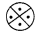Solution:
The distance between the two chord FE = OE + OF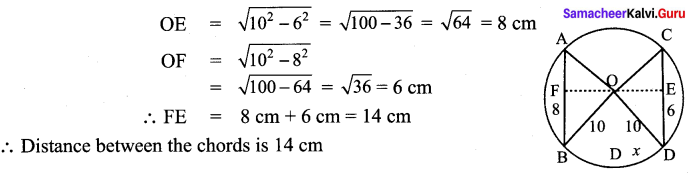∴ Distance between the chords is 14 cm

Question 6.
Two circles of radii 5 cm and 3 cm intersect at two points and the distance between their centres is 4 cm. Find the length of the common chord.
Solution: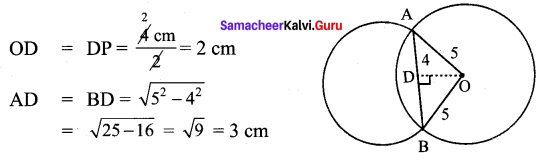The length of the common chord AB = AD + BD = (3 + 3) cm = 6 cm

Question 7.
Find the value of x° in the following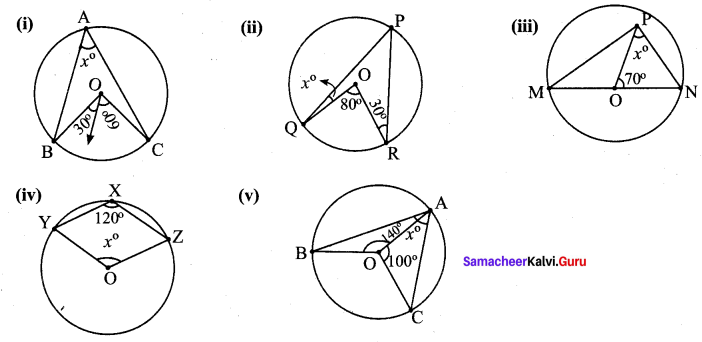Solution: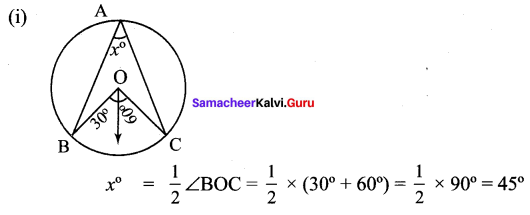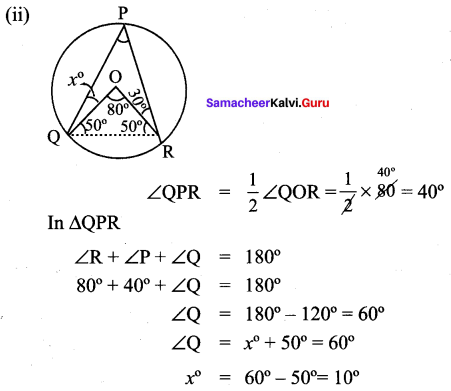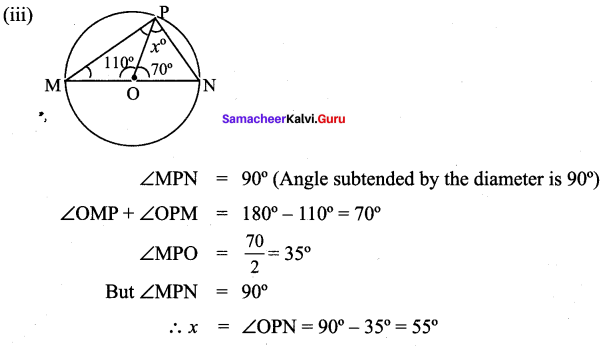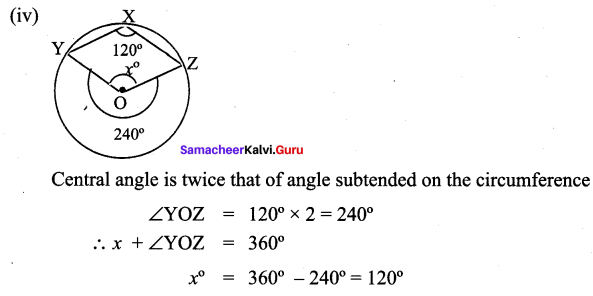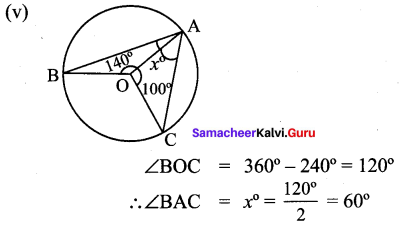Question 8.
In the given figure, ∠CAB = 25°, find ∠BDC, ∠DBA and ∠COB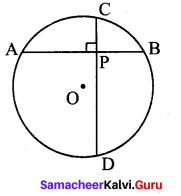Solution: